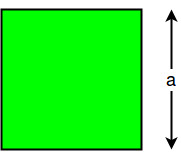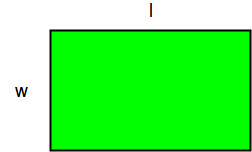# Program to find Perimeter / Circumference of Square and Rectangle

• Last Updated : 06 Apr, 2021

The circumference of a figure is the sum of all the side lengths. To calculate the circumference of square, length of one of the side is required as all sides are equal. To calculate the circumference of rectangle, length and breadth of rectangle is required.
Circumference of a Square:Attention reader! All those who say programming isn't for kids, just haven't met the right mentors yet. Join the  Demo Class for First Step to Coding Coursespecifically designed for students of class 8 to 12.

The students will get to learn more about the world of programming in these free classes which will definitely help them in making a wise career choice in the future.

The circumference of a square is given by the formula:

```C = 4 * a
where a is the side length.```

Examples :

```input: 4
output: 16

input: 3
output: 12```

## C++

 `// CPP program to find``// Circumference of a square``#include ``using` `namespace` `std;` `int` `Circumference(``int` `a)``{``    ``return` `4 * a;``}` `// Driver Code``int` `main()``{``    ``int` `a = 5;``    ``cout << ``"Circumference of"``         ``<<``" a square is "``         ``<< Circumference(a);``    ``return` `0;``}` `// This code is contributed``// by mohitw16`

## Java

 `// Java program to find``// Circumference of a square` `import` `java.io.*;``class` `GFG``{``    ``int` `Circumference(``int` `a)``    ``{``        ``return` `4` `* a;``    ``}` `    ``// Driver code``    ``public` `static` `void` `main(String args[])``    ``{``        ``GFG obj = ``new` `GFG();``        ``int` `a = ``5``;``        ``System.out.println(``"Circumference of "` `+``                                ``"a square is "` `+``                          ``obj.Circumference(a));``    ``}``}` `// This code is contributed``// by Anshika Goyal.`

## Python3

 `# Python3 Program to find``# Circumference of a square` `def` `Circumference(a):``        ``return` `(``4` `*` `a)` `# Driver code``a ``=` `5``c ``=` `Circumference(a)``print``(``"Circumference of a "` `+``       ``"square is % d"` `%` `(c))`

## C#

 `// C# program to find Circumference``// of a square``using` `System;` `class` `GFG``{` `    ``static` `int` `Circumference(``int` `a)``    ``{``        ``return` `4 * a;``    ``}` `    ``// Driver Code``    ``public` `static` `void` `Main()``    ``{``        ``int` `a = 5;` `        ``Console.WriteLine(``"Circumference"` `+``                       ``" of a square is "` `+``                         ``Circumference(a));``    ``}``}` `// This code is contributed by vt_m.`

## PHP

 ``

## Javascript

 ``

Output :

`Circumference Of a square is 20`

Circumference of a rectangle:The circumference of a rectangle is given by the formula:

```C = 2 * (l + W)
where l is the length and W is the width.```

Examples :

```input: 2 4
output: 12

input: 4 6
output: 20```

## C++

 `// C++ Program to find``// Circumference of a rectangle``#include ``using` `namespace` `std;` `int` `Circumference(``int` `l, ``int` `w)``{``    ``return` `(2 * (l + w));``}` `// Driver code``int` `main()``{``    ``int` `l = 8, w = 4;` `    ``int` `c = Circumference(l, w);` `    ``cout << ``"Circumference of a"``         ``<< ``" rectangle is "``         ``<< c << endl;` `    ``return` `0;``}` `// This code is contributed by vt_m.`

## Python3

 `# Python Program to find``# Circumference of a rectangle` `def` `Circumference(l, w):``        ``return` `(``2` `*` `(l ``+` `w))` `# Driver code``l ``=` `8``w ``=` `4``c ``=` `Circumference(l, w)``print``(``"Circumference of a"` `+``  ``" rectangle is % d"` `%` `(c))`

## Java

 `// java Program to find``// Circumference of a rectangle``import` `java.io.*;` `class` `GFG``{` `    ``static` `int` `Circumference(``int` `l,``                             ``int` `w)``    ``{``        ``return` `(``2` `* (l + w));``    ``}` `    ``// Driver code``    ``static` `public` `void` `main(String[] args)``    ``{``        ``int` `l = ``8``, w = ``4``;` `        ``int` `c = Circumference(l, w);` `        ``System.out.println(``"Circumference of "` `+``                         ``"a rectangle is "` `+ c);``    ``}``}` `// This code is contributed by vt_m.`

## C#

 `// C# Program to find``// circumference of a rectangle``using` `System;` `class` `GFG``{` `    ``static` `int` `Circumference(``int` `l,``                             ``int` `w)``    ``{``        ``return` `(2 * (l + w));``    ``}` `    ``// Driver code``    ``static` `public` `void` `Main()``    ``{``        ``int` `l = 8, w = 4;` `        ``int` `c = Circumference(l, w);` `        ``Console.WriteLine(``"Circumference of "` `+``                        ``"a rectangle is "` `+ c);``    ``}``}` `// This code is contributed by vt_m.`

## PHP

 ``

## Javascript

 ``

Output :

`Circumference of a rectangle is 24`

My Personal Notes arrow_drop_up